# 量子计算与量子信息基础

## 1 量子力学基础

### 1.1 什么是量子力学？

Quantum mechanics is a mathematical framework or set of rules for the construction of physical theories.

### 1.2 量子力学的结构

• 描述一个封闭系统的量子状态：状态向量和状态空间(Hilbert space)
• 描述量子状态随时间的变换：酉变换(Unitary transformation)
• 描述对量子系统所进行的测量：投影测量(Measurement)
• 描述一个复合系统的量子状态：张量积(Tensor product of components)

#### 1.2.1 状态空间

$|\alpha|^2+|\beta|^2=1$

$|\psi\rangle=\dbinom{\alpha}{\beta}$

#### 1.2.2 状态演化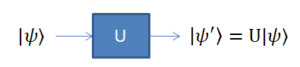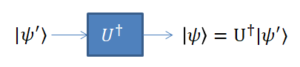The unitarity constraint is the only constraint on quantum gates; any unitary matrix specifies a valid quantum gate.

Pauli门
$I=\begin{bmatrix}1 & 0 \\0 & 1\end{bmatrix},X=\begin{bmatrix}0 & 1\\1 & 0\end{bmatrix},Y=\begin{bmatrix}0 & -i \\i & 0\end{bmatrix},Z=\begin{bmatrix}1 & 0 \\0 & -1\end{bmatrix}$$H|0\rangle=\frac{|0\rangle+|1\rangle}{\sqrt{2}},H|1\rangle=\frac{|0\rangle-|1\rangle}{\sqrt{2}},H=\frac{1}{\sqrt{2}}\begin{bmatrix}1 & 1 \\1 & -1\end{bmatrix}$
$H^2=I, H=(X+Z)/\sqrt(2)$
$HXH=Z, HZH=X, HYH=-Y$

$|00\rangle\to|00\rangle, |01\rangle\to|01\rangle$
$|10\rangle\to|11\rangle, |11\rangle\to|10\rangle$
$|x,y\rangle\to|x,y\bigoplus x\rangle$ ($\bigoplus$: 模2加运算, 此处可以看作异或)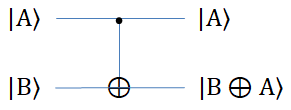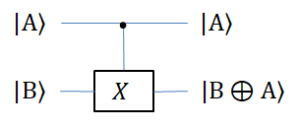CNOT门的矩阵表示
$U_{CNOT}=\begin{bmatrix}1 & 0 & 0 & 0 \\0 & 1 & 0 & 0 \\0 & 0 & 0 & 1\\0 & 0 & 1 & 0\end{bmatrix}$

#### 1.2.3 量子测量

• 结果为0的概率为 $|\alpha|^2$,
• 结果为1的概率为 $|\beta|^2$.

• 测得结果为 $|+\rangle$ 的概率为 $\frac{1}{2}(\alpha+\beta)^2$;
• 测得结果为 $|-\rangle$ 的概率为 $\frac{1}{2}(\alpha-\beta)^2$.

#### 1.2.4 复合系统

$2-qubit$ 状态空间 ($C^2\otimes C^2=C^4$)

$k-qbit$ 是一个 $2^k$ 维的量子系统, 可以由 $k$ 个量子比特的张量积构成.

$\alpha|00\rangle + \beta|01\rangle+\gamma|10\rangle + \delta|11\rangle$$|\alpha|^2+|\beta|^2+|\gamma|^2+|\delta|^2=1$

### 1.3 贝尔态(Bell state)/EPR pair

$|B_{00}\rangle=\frac{1}{\sqrt{2}}(|00\rangle+|11\rangle), |B_{01}\rangle=\frac{1}{\sqrt{2}}(|01\rangle+|10\rangle)$$|B_{10}\rangle=\frac{1}{\sqrt{2}}(|00\rangle-|11\rangle), |B_{11}\rangle=\frac{1}{\sqrt{2}}(|01\rangle-|10\rangle)$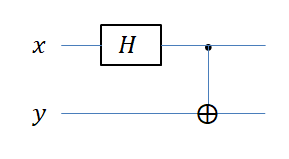$\mid 00\ \rangle$ $\mid B_{00}\ \rangle=\frac{1}{\sqrt{2}}(\mid 00\ \rangle+\mid 11\ \rangle)$
$\mid 01\ \rangle$ $\mid B_{01}\ \rangle=\frac{1}{\sqrt{2}}(\mid 01\ \rangle+\mid 10\ \rangle)$
$\mid10\ \rangle$ $\mid B_{10}\ \rangle=\frac{1}{\sqrt{2}}(\mid 00\ \rangle- \mid 11\ \rangle)$
$\mid 11\ \rangle$ $\mid B_{11}\ \rangle=\frac{1}{\sqrt{2}}(\mid 01\ \rangle-\mid 10\ \rangle)$

### 1.4 Quantum teleportation

• Alice有一个处于叠加态 $|\psi\rangle=\alpha|0\rangle+\beta|1\rangle$ 的量子比特. 她想把该状态发送给在远处的Bob.
• Alice和Bob之间不允许直接进行量子比特传输, 但是Alice和Bob之间共享一个EPR对 $|B_{00}\rangle$

• Step 0: 系统的初始状态为$|\psi_0\rangle=|\psi\rangle|B_{00}\rangle=\frac{1}{\sqrt{2}}(\alpha|0\rangle+\beta|1\rangle)(|00\rangle+|11\rangle)$, 其中前两个qubit属于Alice, 最后一个qubit属于Bob.
• Step 1: Alice首先用CNOT门作用于她的两个qubit, 从而状态变为$|\psi_1\rangle=\frac{\alpha}{\sqrt{2}}|0\rangle(|00\rangle+|11\rangle)+\frac{\beta}{\sqrt{2}}|1\rangle(|10\rangle+|01\rangle)$.
• Step 2: Alice用Hadamard门作用于第一个qubit, 从而得到 $|\psi_2\rangle\propto(|00\rangle|\psi\rangle+|01\rangle X|\psi\rangle+|10\rangle Z|\psi\rangle+|11\rangle XZ|\psi\rangle)$.
• Step 3: Alice对她的两个qubit做测量, 然后告诉Bob测量结果, 例如, 如果测量得到 $|01\rangle$, 则 $|\psi_3\rangle=|01\rangle X|\psi\rangle$.
• Step 4: 由于Bob获取了测量结果, 从而他可以用酉矩阵对量子态进行纠正. 在上面的例子中, $X(X|\psi\rangle)=X^2|\psi\rangle=|\psi\rangle$, 也就是说, Bob最终可以接收到 $|\psi_4\rangle=|01\rangle|\psi\rangle$.

Teleportation 不能实现超光速通信, 因为为了完成teleportation, Alice必须通过经典通信信道发送她的测量结果给Bob.

## 2 量子密码基础

### 2.1 量子密钥分发(Quantum key distribution, QKD)

QKD利用量子力学中的不确定性原则作为密码学的基础. 在传统的信息论和密码学中, 数字通信原则上是会受到被动地监听和复制的. 然而, 当信息被以非正交量子态(如偏振方向为0°, 45°, 90° 和135°的单光子)编码后, 窃听者在不知道传输过程中使用的密钥的情况下, 原则上不能可靠地读取或复制. 窃听者也不能在获取部分信息的同时不以随机不可控的方式改变它(这会被合法用户发现)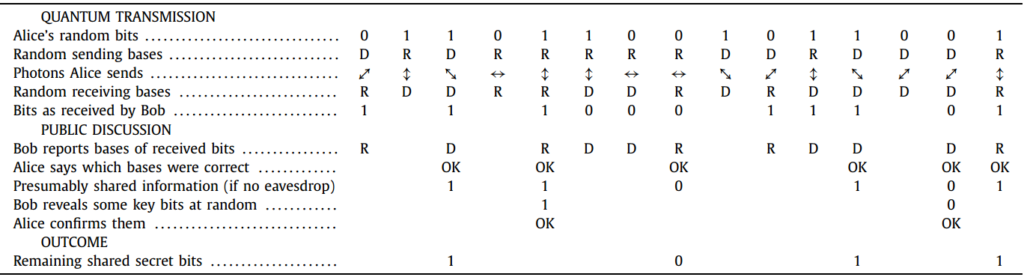### 2.2 量子比特承诺

• 承诺阶段: Alice向Bob承诺一个比特b, 要求: Bob无法知道b的信息(隐蔽性)
• 打开阶段: Alice向Bob正式她在第一阶段承诺的确实是b, 但Alice无法欺骗Bob, 即不能篡改b的值(约束性)

[BB84] 提出的量子比特承诺协议

• Alice和Bob首先协商一个安全参数 $s$
• 在承诺阶段, 若Alice承诺的是 $b=0$, 则她制备 $s$ 个处于 $z$ 基基态的单光子, 即 $|0\rangle$ 态或 $|1\rangle$ 态; 反之若 $b=1$, 则制备 $s$ 个处于 $x$ 基基态的单光子, 即 $|+\rangle$ 态或 $|-\rangle$ 态. 最后将这 $s$ 个单光子发送给Bob. Bob收到这 $s$ 个单光子后, 随机选择 $z$ 基或 $x$ 基测量, 并记录测量结果.
• 在打开阶段, Alice告诉Bob她的承诺 $b$ 和每个光子所处的量子态. Bob根据选择正确测量基的一半光子的测量结果判断Alice是否说谎. 若无, Bob相信Alice之前的承诺.

### 2.3 量子秘密共享(Quantum Secret Sharing, QSS)

QSS根据共享信息的不同, 可分为共享经典/量子信息两类.

#### 2.3.1 KKI协议

KKI使用两粒子纠缠态实现QSS, 其安全性基于非正交态不可区分性.

The state generated from a type-II parametric down-conversion crystal: $|\psi\rangle=\frac{1}{\sqrt{2}}(|z+\rangle_A|z-\rangle_B+e^{i\alpha}|z-\rangle_A|z+\rangle_B)$

• $\alpha$: 晶体的双折射相移(birefringent phase shift of the crystal)
• $|z+\rangle$, $|z-\rangle$: 自旋本征态(spin eigenstate)或水平和垂直偏振本征态(horizontal and vertical polarization eigenstates)
• A, B: Alice and Bob

$|\phi^{\pm}\rangle=\frac{1}{\sqrt{2}}(|z+\rangle_A|z+\rangle_B\pm|z-\rangle_A|z-\rangle_B$$|\psi^{\pm}\rangle=\frac{1}{\sqrt{2}}(|z+\rangle_A|z-\rangle_B\pm|z-\rangle_A|z+\rangle_B$

$|x+\rangle=\frac{1}{\sqrt{2}}(|z+\rangle+|z-\rangle), |x-\rangle=\frac{1}{\sqrt{2}}(|z+\rangle-|z-\rangle)$

$|\phi^+\rangle=\frac{1}{\sqrt{2}}(|x+\rangle_A|x+\rangle_B+|x-\rangle_A|x-\rangle_B)$$|\phi^-\rangle=\frac{1}{\sqrt{2}}(|x+\rangle_A|x-\rangle_B+|x-\rangle_A|x+\rangle_B)$$|\psi^+\rangle=\frac{1}{\sqrt{2}}(|x+\rangle_A|x+\rangle_B-|x-\rangle_A|x-\rangle_B)$$|\psi^+\rangle=\frac{1}{\sqrt{2}}(|x-\rangle_A|x+\rangle_B-|x+\rangle_A|x-\rangle_B)$

$|\Psi^+\rangle\equiv\frac{1}{\sqrt{2}}(|\phi^-\rangle+|\psi^+\rangle)$$=\frac{1}{\sqrt{2}}(|z+\rangle_A|x+\rangle_B+|z-\rangle_A|x-\rangle_A)$$=\frac{1}{\sqrt{2}}(|x+\rangle_A|z+\rangle_B+|x-\rangle_A|z-\rangle_B)$

$|\langle\psi^+|\Psi^+\rangle|^2=|\langle\psi^+|\Phi\rangle|^2=\frac{1}{2}, |\langle\phi^-|\Psi^+\rangle|^2=|\langle\phi^+|\Phi^-\rangle|^2=\frac{1}{2}$

• 发送者选择状态${|0\rangle,|1\rangle}\Leftrightarrow{|\psi^+\rangle,|\phi^-\rangle}$, 对二进制随机比特串 $S$ 做编码.
• Alice和Bob分别接收到一种粒子(光子), 然后随机用 $z$$x$ 基进行测量.
• 发送者随机选择部分粒子进行窃听检测: Alice和Bob先公开测量结果, 再公开测量基(且先公开结果的后公开基).
• 发送者根据初始态计算错误率, 如果错误率足够低则继续.
• Alice和Bob公开其余粒子的测量基, 发送者公开初始基, 以一半的概率, Alice和Bob的测量结果与发送者的初始态具有关联, 因此Alice和Bob合作可以推出发送者的量子态.©️2019 CSDN 皮肤主题: 书香水墨 设计师: CSDN官方博客